# RD Sharma Solutions for Class 6 Chapter 7: Decimals Exercise 7.3

The students who aspire to score well in the exam can make use of RD Sharma Solutions for Class 6. The exercise 7.3 of chapter 7 has problems which help to determine the hundredths and thousandths place in the given set of questions. These problems are answered by experts with explanations in simple language to make learning fun for the students. To know more about the concepts which are covered in this exercise, the students can use RD Sharma Solutions for Class 6 Maths Chapter 7 Decimals Exercise 7.3 PDF which are given here.

## RD Sharma Solutions for Class 6 Chapter 7: Decimals Exercise 7.3 Download PDF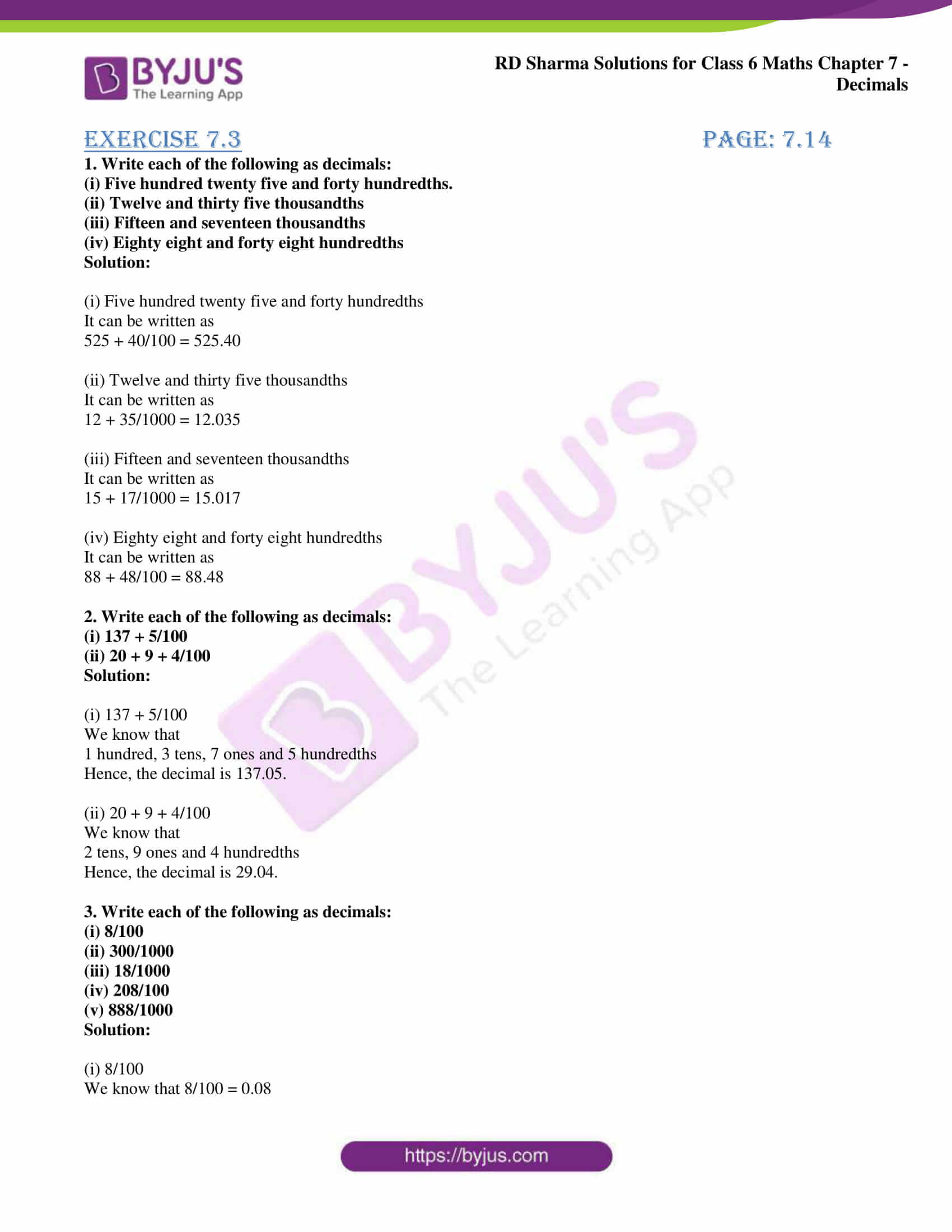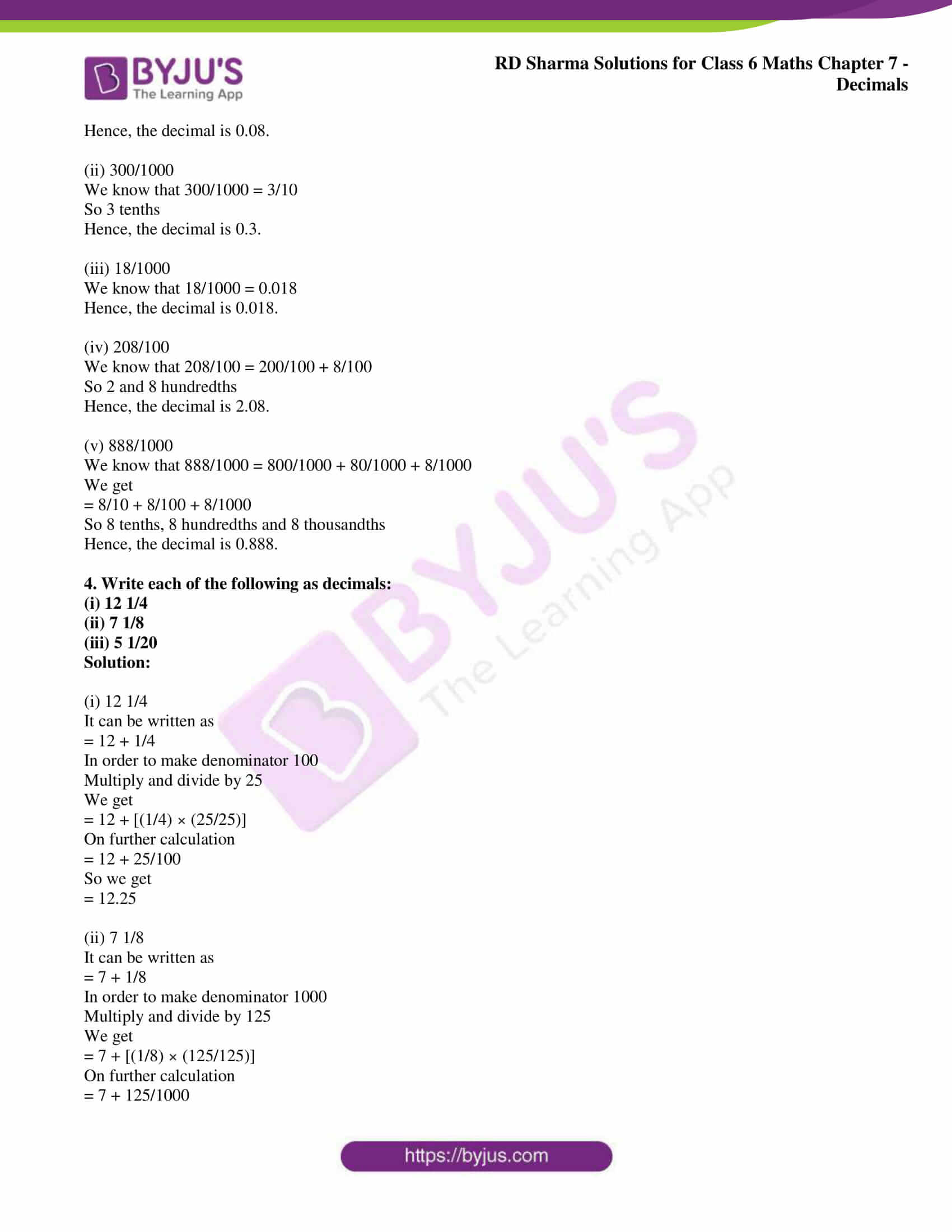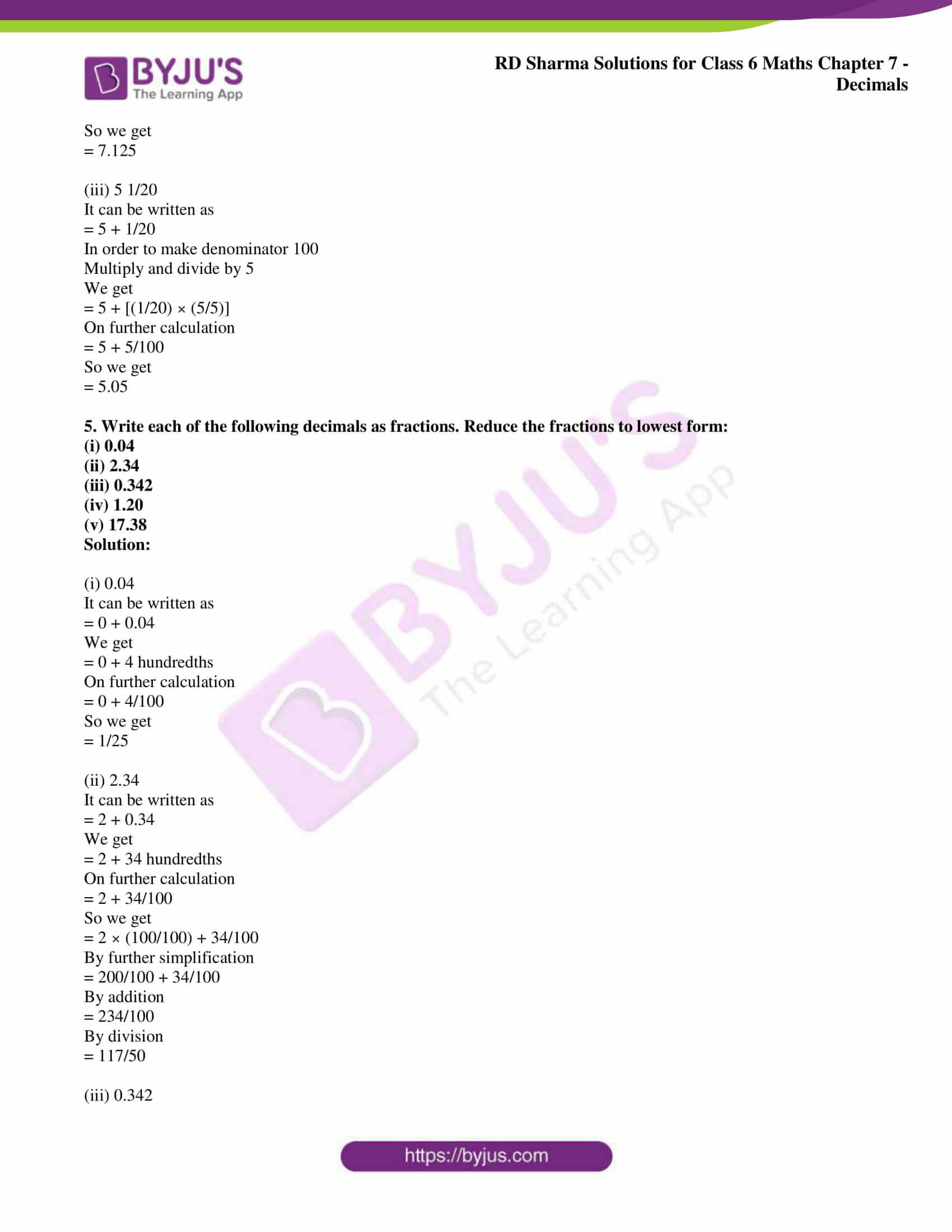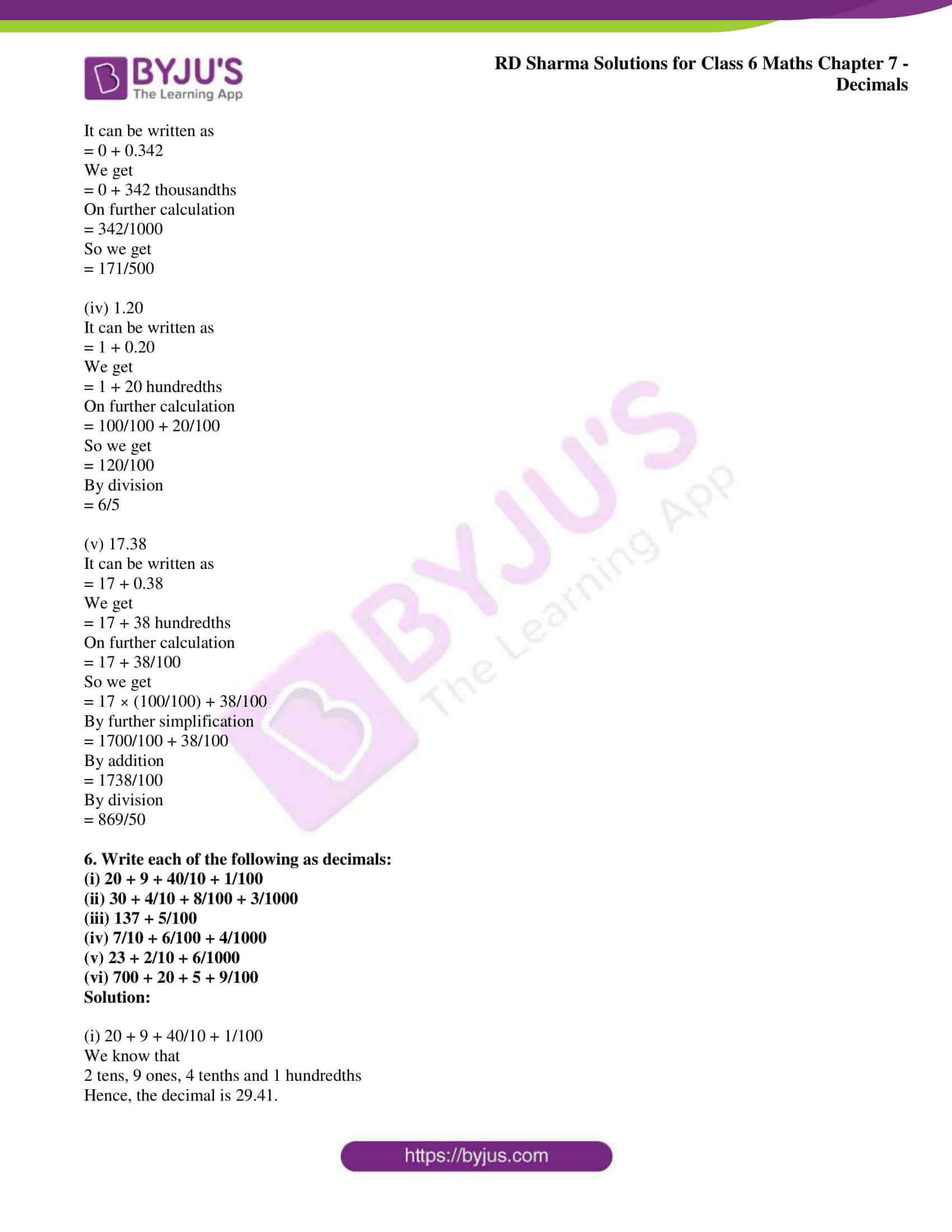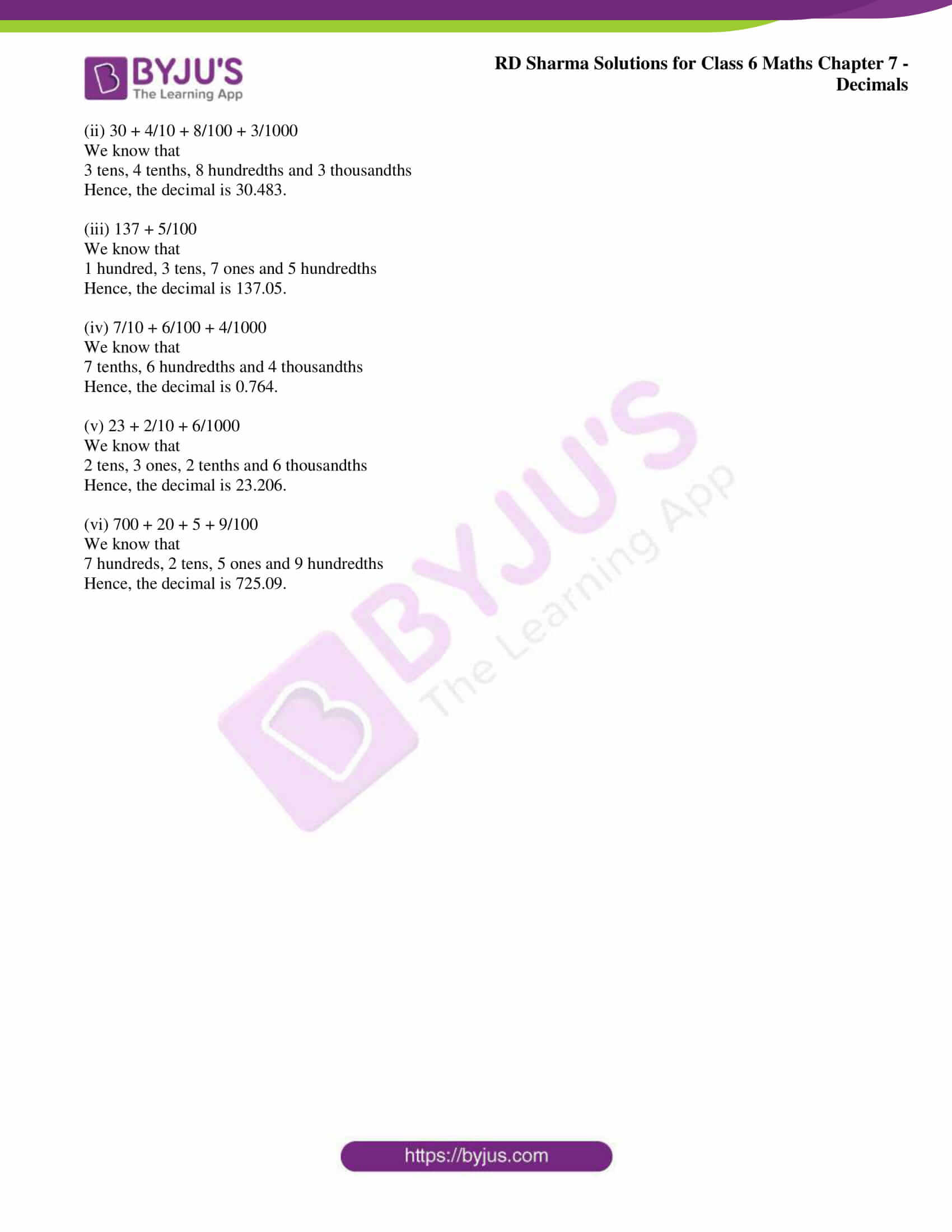### Access RD Sharma Solutions for Class 6 Chapter 7: Decimals Exercise 7.3

1. Write each of the following as decimals:

(i) Five hundred twenty five and forty hundredths.

(ii) Twelve and thirty five thousandths

(iii) Fifteen and seventeen thousandths

(iv) Eighty eight and forty eight hundredths

Solution:

(i) Five hundred twenty five and forty hundredths

It can be written as

525 + 40/100 = 525.40

(ii) Twelve and thirty five thousandths

It can be written as

12 + 35/1000 = 12.035

(iii) Fifteen and seventeen thousandths

It can be written as

15 + 17/1000 = 15.017

(iv) Eighty eight and forty eight hundredths

It can be written as

88 + 48/100 = 88.48

2. Write each of the following as decimals:

(i) 137 + 5/100

(ii) 20 + 9 + 4/100

Solution:

(i) 137 + 5/100

We know that

1 hundred, 3 tens, 7 ones and 5 hundredths

Hence, the decimal is 137.05.

(ii) 20 + 9 + 4/100

We know that

2 tens, 9 ones and 4 hundredths

Hence, the decimal is 29.04.

3. Write each of the following as decimals:

(i) 8/100

(ii) 300/1000

(iii) 18/1000

(iv) 208/100

(v) 888/1000

Solution:

(i) 8/100

We know that 8/100 = 0.08

Hence, the decimal is 0.08.

(ii) 300/1000

We know that 300/1000 = 3/10

So 3 tenths

Hence, the decimal is 0.3.

(iii) 18/1000

We know that 18/1000 = 0.018

Hence, the decimal is 0.018.

(iv) 208/100

We know that 208/100 = 200/100 + 8/100

So 2 and 8 hundredths

Hence, the decimal is 2.08.

(v) 888/1000

We know that 888/1000 = 800/1000 + 80/1000 + 8/1000

We get

= 8/10 + 8/100 + 8/1000

So 8 tenths, 8 hundredths and 8 thousandths

Hence, the decimal is 0.888.

4. Write each of the following as decimals:

(i) 12 1/4

(ii) 7 1/8

(iii) 5 1/20

Solution:

(i) 12 1/4

It can be written as

= 12 + 1/4

In order to make denominator 100

Multiply and divide by 25

We get

= 12 + [(1/4) × (25/25)]

On further calculation

= 12 + 25/100

So we get

= 12.25

(ii) 7 1/8

It can be written as

= 7 + 1/8

In order to make denominator 1000

Multiply and divide by 125

We get

= 7 + [(1/8) × (125/125)]

On further calculation

= 7 + 125/1000

So we get

= 7.125

(iii) 5 1/20

It can be written as

= 5 + 1/20

In order to make denominator 100

Multiply and divide by 5

We get

= 5 + [(1/20) × (5/5)]

On further calculation

= 5 + 5/100

So we get

= 5.05

5. Write each of the following decimals as fractions. Reduce the fractions to lowest form:

(i) 0.04

(ii) 2.34

(iii) 0.342

(iv) 1.20

(v) 17.38

Solution:

(i) 0.04

It can be written as

= 0 + 0.04

We get

= 0 + 4 hundredths

On further calculation

= 0 + 4/100

So we get

= 1/25

(ii) 2.34

It can be written as

= 2 + 0.34

We get

= 2 + 34 hundredths

On further calculation

= 2 + 34/100

So we get

= 2 × (100/100) + 34/100

By further simplification

= 200/100 + 34/100

= 234/100

By division

= 117/50

(iii) 0.342

It can be written as

= 0 + 0.342

We get

= 0 + 342 thousandths

On further calculation

= 342/1000

So we get

= 171/500

(iv) 1.20

It can be written as

= 1 + 0.20

We get

= 1 + 20 hundredths

On further calculation

= 100/100 + 20/100

So we get

= 120/100

By division

= 6/5

(v) 17.38

It can be written as

= 17 + 0.38

We get

= 17 + 38 hundredths

On further calculation

= 17 + 38/100

So we get

= 17 × (100/100) + 38/100

By further simplification

= 1700/100 + 38/100

= 1738/100

By division

= 869/50

6. Write each of the following as decimals:

(i) 20 + 9 + 40/10 + 1/100

(ii) 30 + 4/10 + 8/100 + 3/1000

(iii) 137 + 5/100

(iv) 7/10 + 6/100 + 4/1000

(v) 23 + 2/10 + 6/1000

(vi) 700 + 20 + 5 + 9/100

Solution:

(i) 20 + 9 + 40/10 + 1/100

We know that

2 tens, 9 ones, 4 tenths and 1 hundredths

Hence, the decimal is 29.41.

(ii) 30 + 4/10 + 8/100 + 3/1000

We know that

3 tens, 4 tenths, 8 hundredths and 3 thousandths

Hence, the decimal is 30.483.

(iii) 137 + 5/100

We know that

1 hundred, 3 tens, 7 ones and 5 hundredths

Hence, the decimal is 137.05.

(iv) 7/10 + 6/100 + 4/1000

We know that

7 tenths, 6 hundredths and 4 thousandths

Hence, the decimal is 0.764.

(v) 23 + 2/10 + 6/1000

We know that

2 tens, 3 ones, 2 tenths and 6 thousandths

Hence, the decimal is 23.206.

(vi) 700 + 20 + 5 + 9/100

We know that

7 hundreds, 2 tens, 5 ones and 9 hundredths

Hence, the decimal is 725.09.

#### 1 Comment

1. Rishabh Agarwal